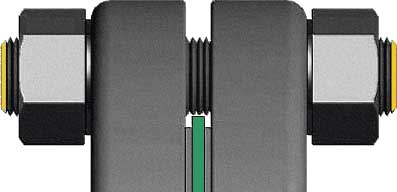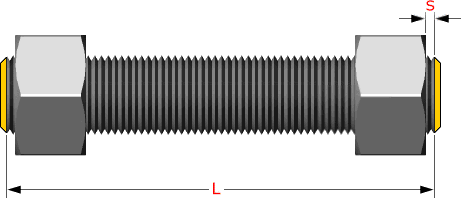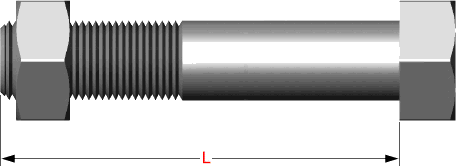# Method for calculating Stud Bolt length

## The Stud Bolt theoretical length can be calculated by means of the formula..

L = 2 (s + n + h + rf) + gs = free threads (equals 1/3 time bolt diameter)
n = nut height (equals nominal bolt diameter)
h = flange (plate) thickness
rf = height of raised face

#### The height of a nut for a Stud Bolt is the same as the diameter of the thread rod

Studs are measured parallel to the axis (L) from the first to the thread without the chamfers (points). S = free threads equals 1/3 time bolt diaHex bolts are measured from under the head to the top of de boltExplore the World of Piping